|

# 智臻至诚 尽享奢华TOTO带你体验不一样的卫浴之旅

今年是中国国际厨房、卫浴设施展走过的第23个年头了，本次展会上，TOTO携新品惊艳亮相。这一次，TOTO给大家带来了一场别开生面的“SMART TRIP”智臻之旅；再次刷新了我们对卫浴产品的认知，给观众展示了全新的智能化卫浴产品、新理念、新设计。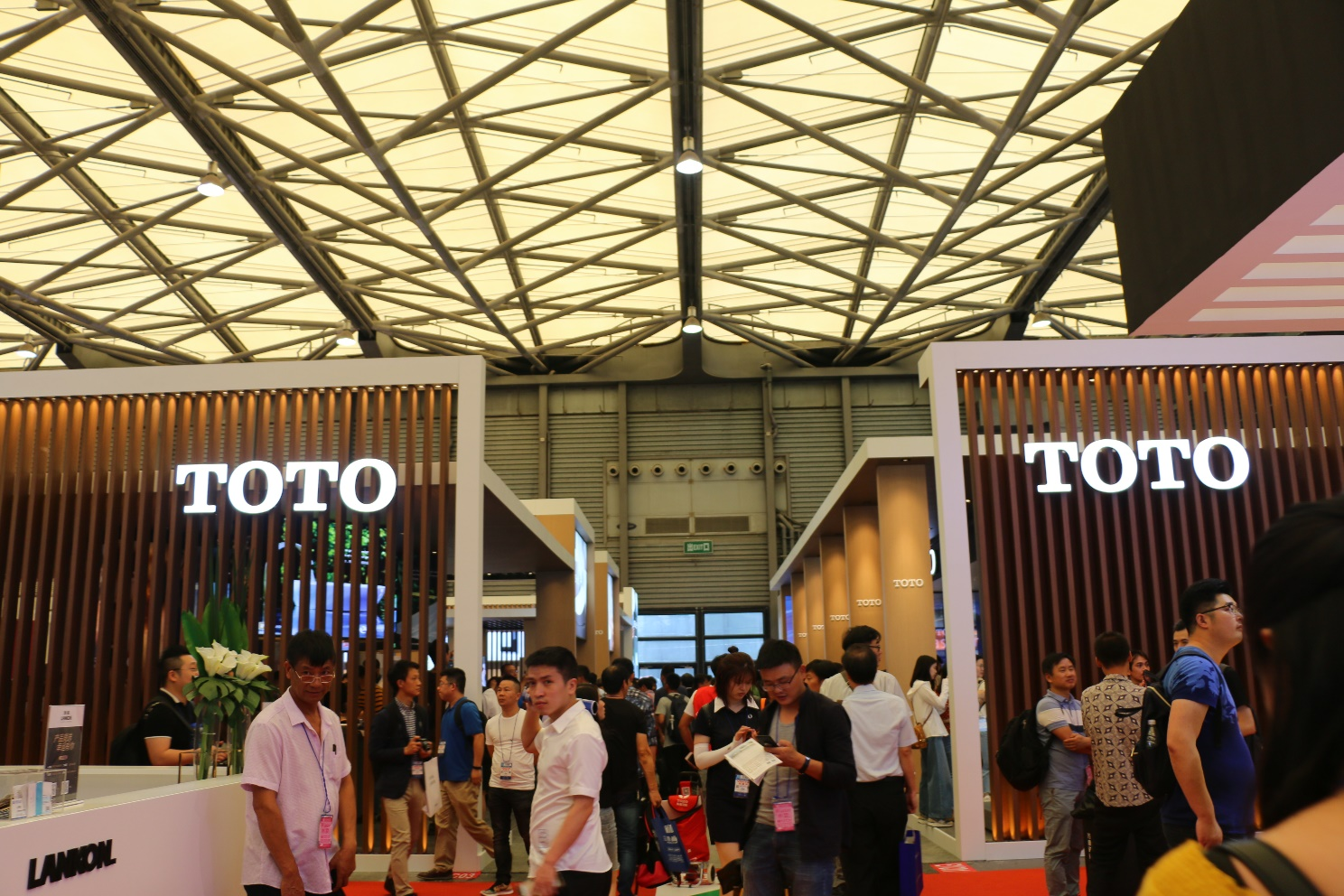诺锐斯特 智臻奢华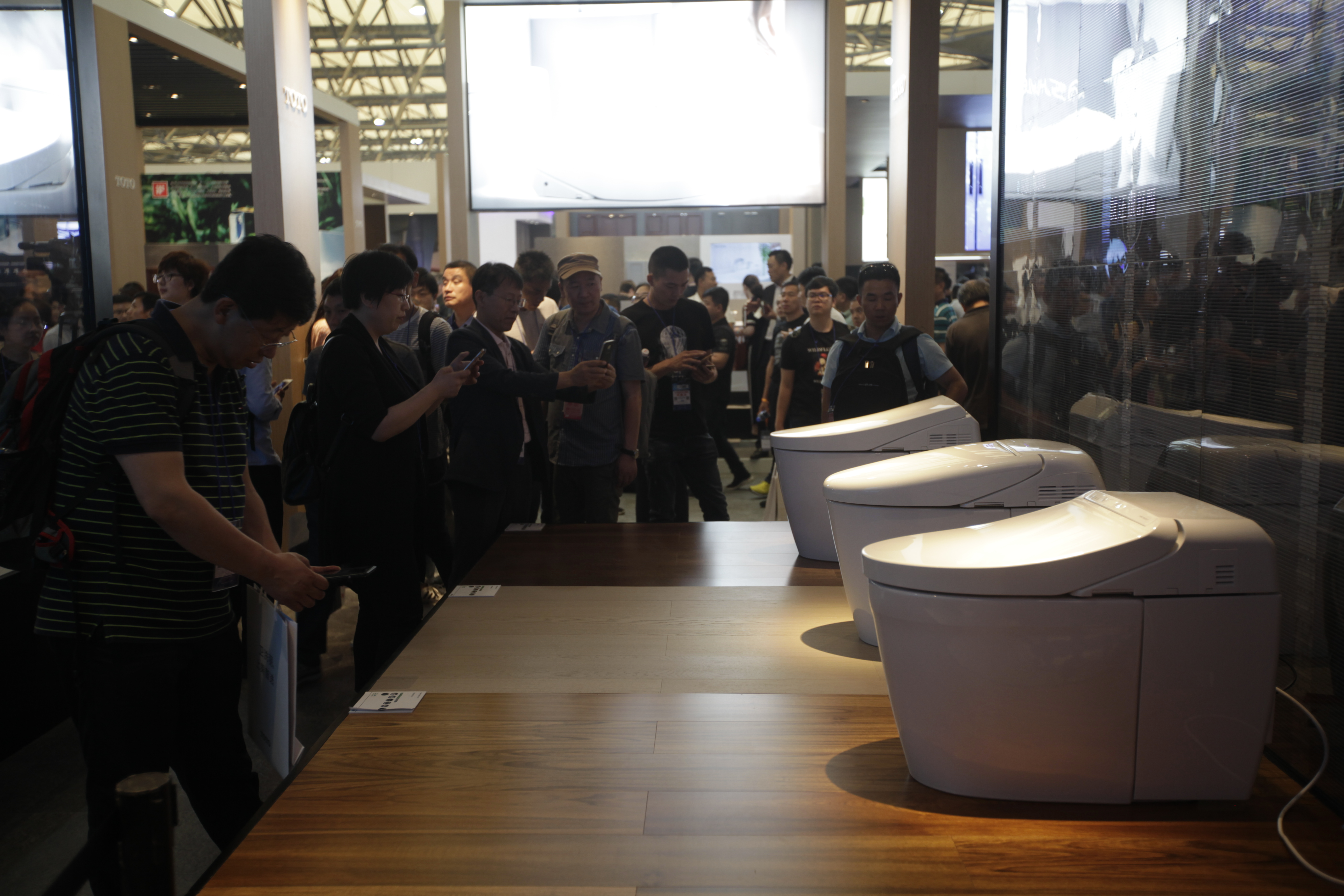明星产品诺锐斯特系列一直都是TOTO高端智能产品的代表；优雅高贵的造型和人性化的智能体验，让产品仿佛是一款艺术品，让卫浴空房间增色。厨卫展期间，TOTO推出了新近研发的诺锐斯特坐便器AH、RH、DH，这些产品柔和雅致、简洁时尚，可以为空间搭配提供更多选择。诺锐斯特坐便器不仅拥有精致的外观，还拥有可圈可点的功能。自动开闭、冲洗这些基本功能不在话下，超漩、智洁、便前喷雾、无棱内壁设计等功能让产品更贴近生活、贴近用户的使用体验。诺锐斯特NX坐便器，更具有智炫、智净等功能，将坐便器的清洁水平提升到了新的层次。

WASHLET+上乘智选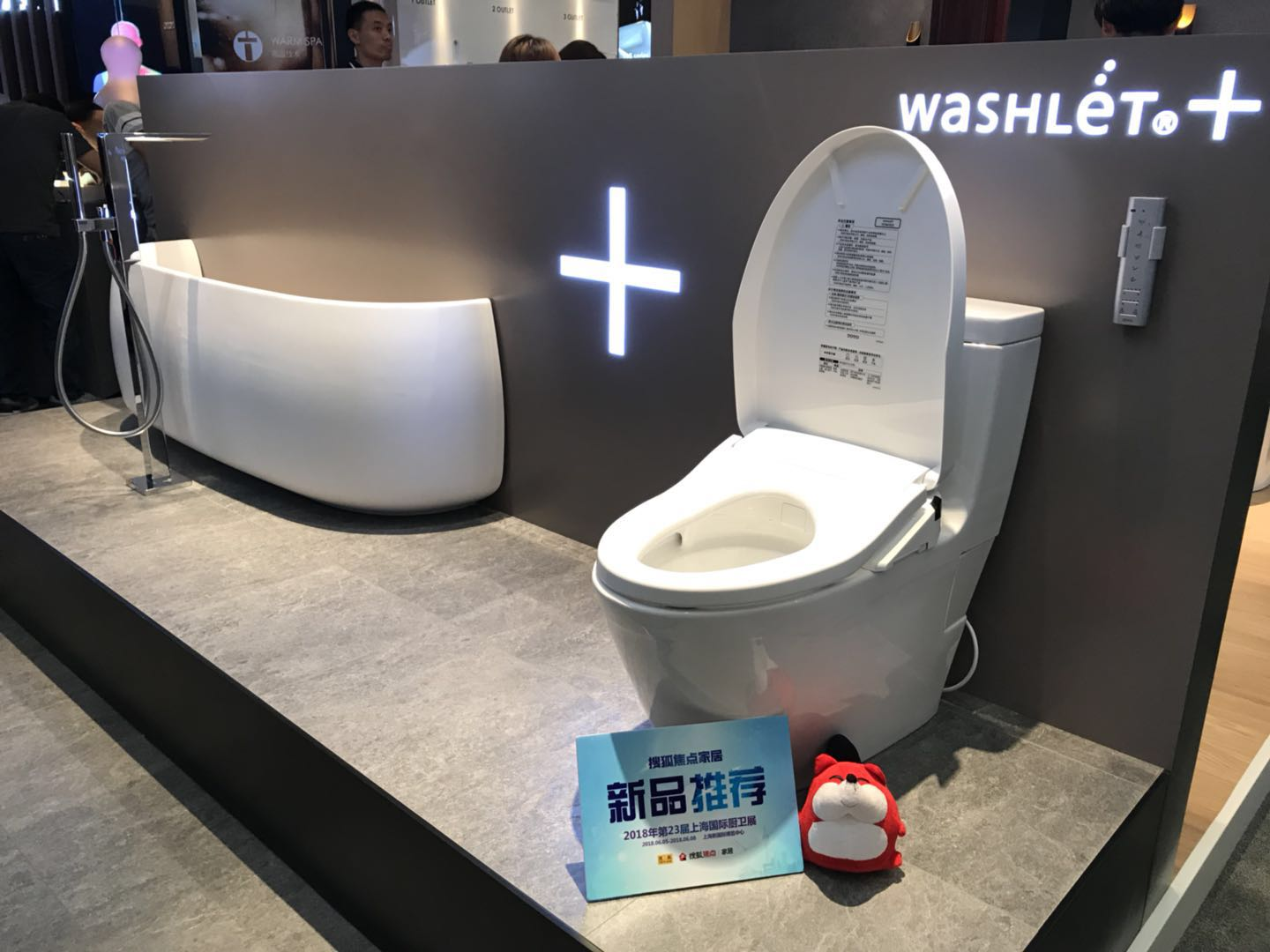“中国游客抢购日本马桶盖”的新闻，让“智能马桶盖”的热度飙升。TOTO作为“智能马桶盖”的先行者，再次引领行业，推出了全新产品——WASHLET+；这是坐便器与卫洗丽组合而成的新系列，大有成为现代生活标配的趋势。

区别于传统的WASHLET安装线与水管外露的造型，WASHLET+系列将这些影响美观度的部件“隐藏”起来，而这一改进，无疑给您的日常生活带来了舒适清洁的新标准。”智能协作，洁净体验“由三种革新的坐便器技术组合而成，智洁的超平滑陶瓷表面，洁净不易留痕。超漩360度强劲水流、实现全方位高效节水冲洗。智净，电解除菌水轻松分解污物，污垢难以残留。尽情享受清洁与舒适赋予的全新生活。自1980年TOTO推出首款卫洗丽以来，目前全球销量已突破4000万台，健康水洗的理念被全世界所接受。现在TOTO又推出了WASHLET+产品，势必又将开启新的卫洗丽热潮。

漂浮浴缸在这里找回自己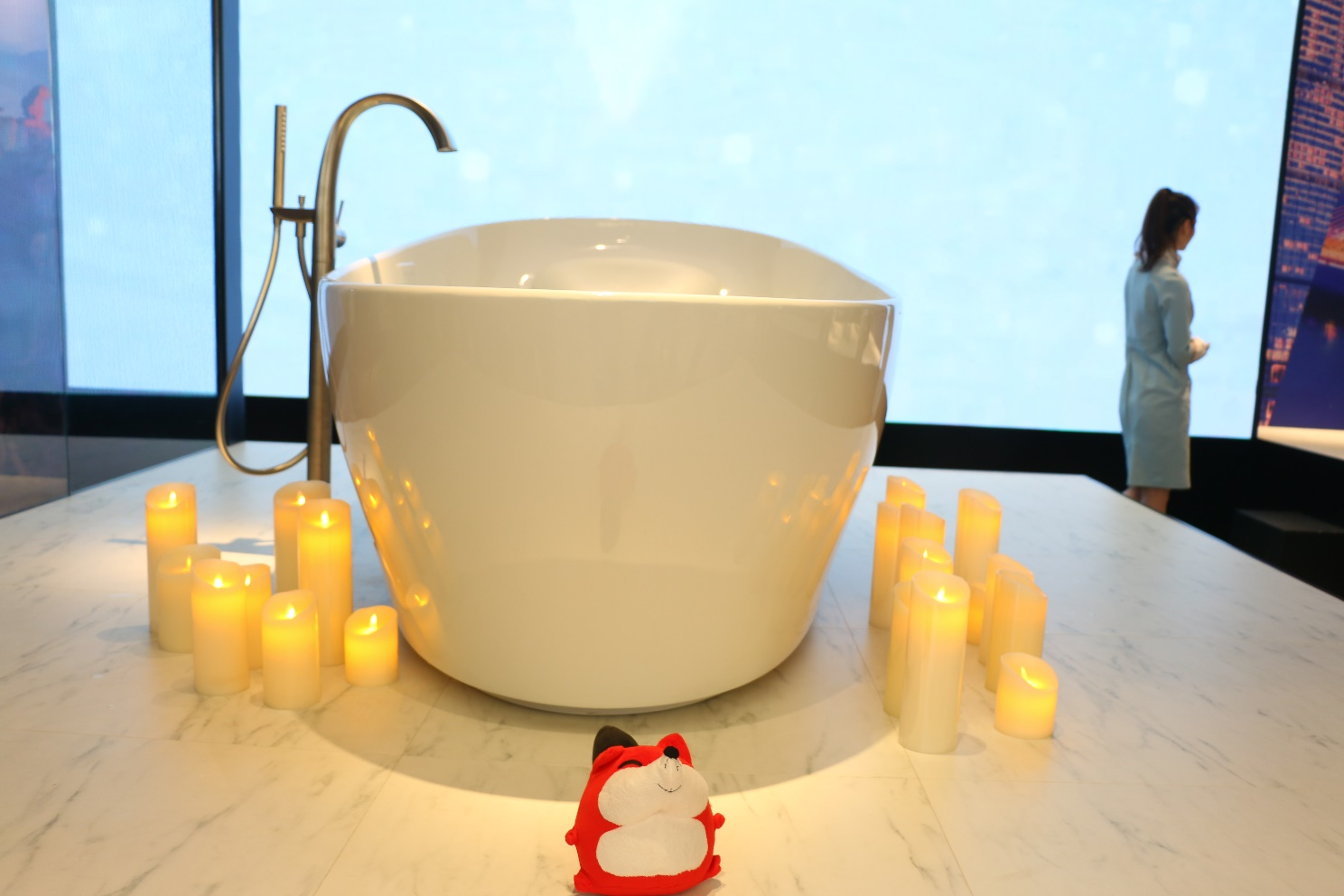曾有一篇网红贴写道：男人下班后回家前喜欢在车里独处10分钟，因为那10分钟他属于自己。一二线城市的快节奏和高压力让很多人在别人的节奏里失去“自我”，自我放松与给他人空间尤为珍贵。

为此，TOTO的“漂浮浴缸”应运而生。采用最新卫浴科技，利用全新的“纤浮”技术，借助水的浮力，让沐浴者感觉处于一种非常稳定的漂浮状态，仿若太空遨游，这时清空脑中一切杂念，如冥想一般。展会展出的新款浴缸，延续了“漂浮浴缸”的设计理念，通过特有的技术，分析捕捉人体在水中的运动机制和活动状态，让人体以舒服的自然姿势，在水中入浴。

全新台盆柜 为您想得更多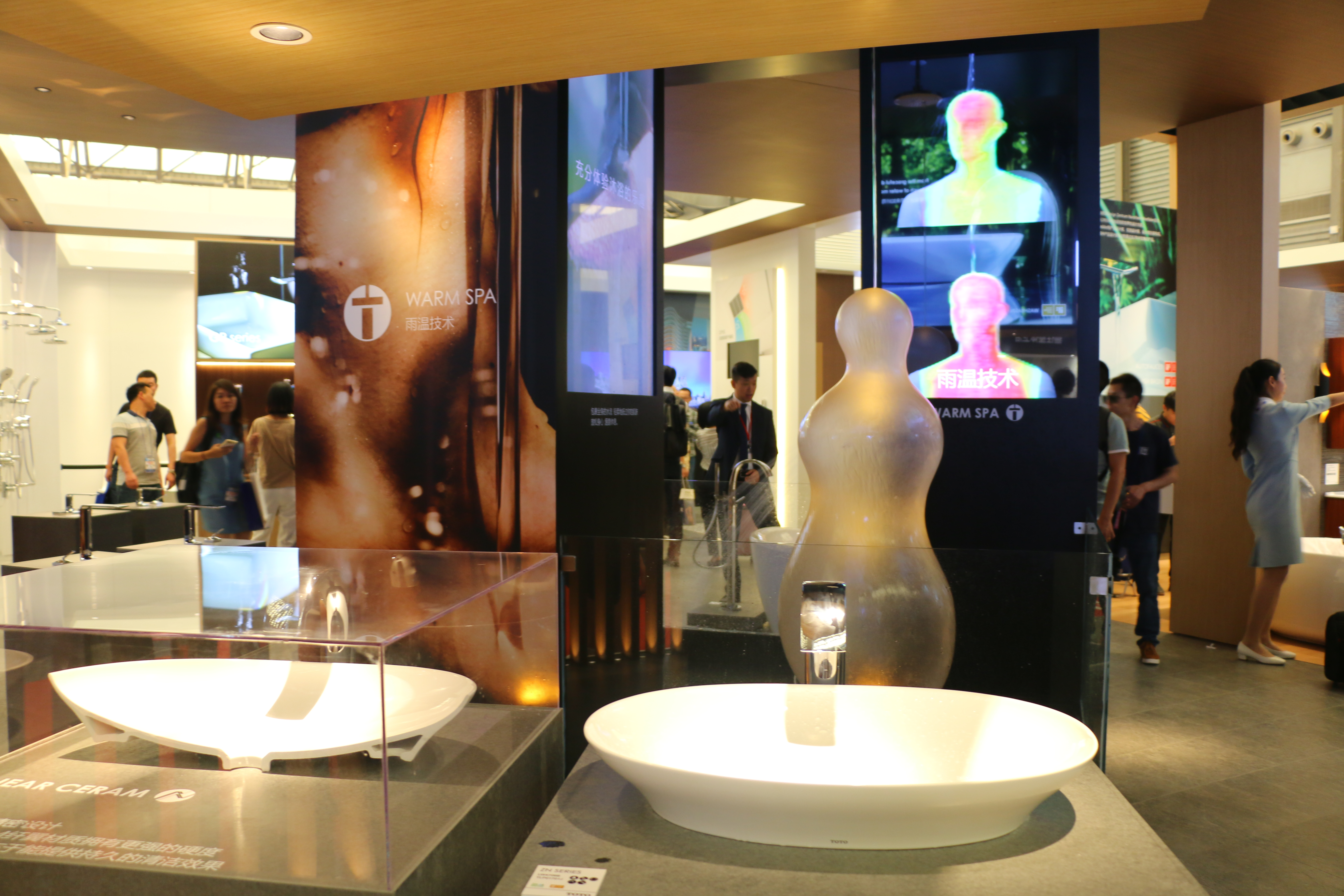在卫浴空间里，台盆柜是利用频率极高的产品之一。本次TOTO推出的新款台盆柜，外观设计上或古典，或现代；在细节方面，也将人性设计发挥到尽致。台盆倾斜式设计，你可以很方便的蓄水清洗小件衣物。抽拉式水嘴设计，便于用水场景自由切换。排水口结构设计，易于取出、清洁，毛发不易堵塞。台盆日本进口SMC材质，坚固不易划伤。镜柜设计也非常人性，各种收纳空间，可放置各类大小不同的生活用品。缓冲式拉门与抽屉设计，不仅关闭静音，也极好地避免产品的碰撞损伤。真正的好产品，就是尽可能，从人性角度出发，考虑使用中的细节，让所有人方便使用，享受便捷。而这也是TOTO设计产品的初衷。

SMART TRIP体验奢华之旅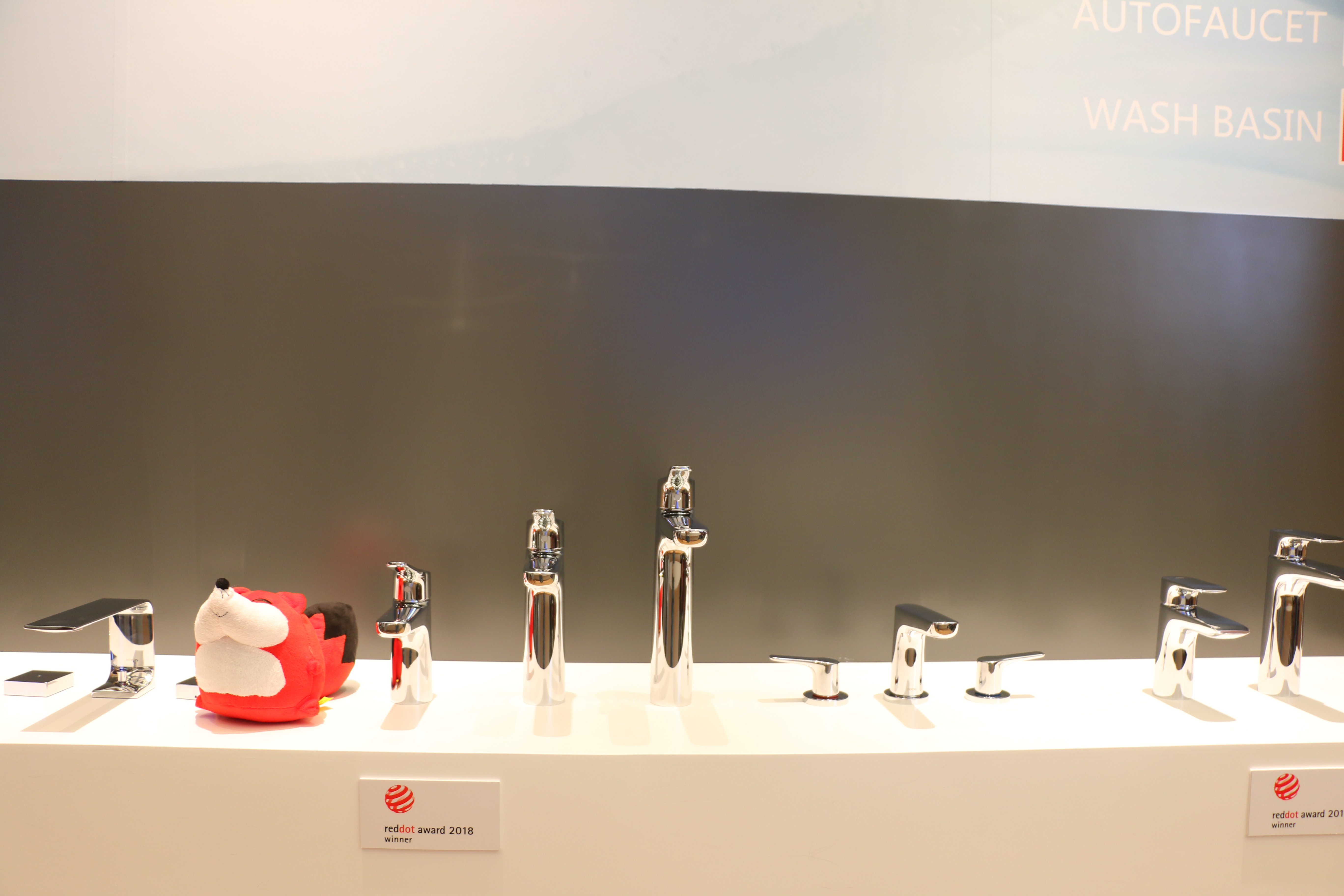一场说走就走的旅行是每个人的梦想，同样，拥有属于自己的SMART TRIP也在逐渐变成我们的理想生活，在生活这场旅途中发现和享受，并将旅行变成日常生活的延续和升级。

作为卫浴生活领导品牌，TOTO对旅行的关注与对消费者生活方式一贯的深刻洞察，让他们在百年的企业历史中，与设计师、开发商等专业领域人士保持了密切的合作。近几十年，TOTO全品类产品早已是诸多国际集团高端奢华酒店品牌的首选之一。今年4月，SMART TRIP平台正式上线，这是一个可消费兑换高端酒店入住体验的在线服务平台，消费者每月都可通过多种渠道参加该活动，赢取免费入住高端酒店的机会，借助这个平台，TOTO旨在让更多的消费者进入这些奢华酒店体验与感受TOTO带来的与众不同的生活。

智臻探寻，从住到行，让感官享受与实用品质结合,在TOTO营造的世界里，生活可以很SMART。

`声明：本文由入驻焦点开放平台的作者撰写，除焦点官方账号外，观点仅代表作者本人，不代表焦点立场错误信息举报电话： 400-099-0099，邮箱：jubao@vip.sohu.com，或点此进行意见反馈，或点此进行举报投诉。`A B C D E F G H J K L M N P Q R S T W X Y Z
A - B - C - D - E
• A
• 鞍山
• 安庆
• 安阳
• 安顺
• 安康
• 澳门
• B
• 北京
• 保定
• 包头
• 巴彦淖尔
• 本溪
• 蚌埠
• 亳州
• 滨州
• 北海
• 百色
• 巴中
• 毕节
• 保山
• 宝鸡
• 白银
• 巴州
• C
• 承德
• 沧州
• 长治
• 赤峰
• 朝阳
• 长春
• 常州
• 滁州
• 池州
• 长沙
• 常德
• 郴州
• 潮州
• 崇左
• 重庆
• 成都
• 楚雄
• 昌都
• 慈溪
• 常熟
• D
• 大同
• 大连
• 丹东
• 大庆
• 东营
• 德州
• 东莞
• 德阳
• 达州
• 大理
• 德宏
• 定西
• 儋州
• 东平
• E
• 鄂尔多斯
• 鄂州
• 恩施
F - G - H - I - J
• F
• 抚顺
• 阜新
• 阜阳
• 福州
• 抚州
• 佛山
• 防城港
• G
• 赣州
• 广州
• 桂林
• 贵港
• 广元
• 广安
• 贵阳
• 固原
• H
• 邯郸
• 衡水
• 呼和浩特
• 呼伦贝尔
• 葫芦岛
• 哈尔滨
• 黑河
• 淮安
• 杭州
• 湖州
• 合肥
• 淮南
• 淮北
• 黄山
• 菏泽
• 鹤壁
• 黄石
• 黄冈
• 衡阳
• 怀化
• 惠州
• 河源
• 贺州
• 河池
• 海口
• 红河
• 汉中
• 海东
• 怀来
• I
• J
• 晋中
• 锦州
• 吉林
• 鸡西
• 佳木斯
• 嘉兴
• 金华
• 景德镇
• 九江
• 吉安
• 济南
• 济宁
• 焦作
• 荆门
• 荆州
• 江门
• 揭阳
• 金昌
• 酒泉
• 嘉峪关
K - L - M - N - P
• K
• 开封
• 昆明
• 昆山
• L
• 廊坊
• 临汾
• 辽阳
• 连云港
• 丽水
• 六安
• 龙岩
• 莱芜
• 临沂
• 聊城
• 洛阳
• 漯河
• 娄底
• 柳州
• 来宾
• 泸州
• 乐山
• 六盘水
• 丽江
• 临沧
• 拉萨
• 林芝
• 兰州
• 陇南
• M
• 牡丹江
• 马鞍山
• 茂名
• 梅州
• 绵阳
• 眉山
• N
• 南京
• 南通
• 宁波
• 南平
• 宁德
• 南昌
• 南阳
• 南宁
• 内江
• 南充
• P
• 盘锦
• 莆田
• 平顶山
• 濮阳
• 攀枝花
• 普洱
• 平凉
Q - R - S - T - W
• Q
• 秦皇岛
• 齐齐哈尔
• 衢州
• 泉州
• 青岛
• 清远
• 钦州
• 黔南
• 曲靖
• 庆阳
• R
• 日照
• 日喀则
• S
• 石家庄
• 沈阳
• 双鸭山
• 绥化
• 上海
• 苏州
• 宿迁
• 绍兴
• 宿州
• 三明
• 上饶
• 三门峡
• 商丘
• 十堰
• 随州
• 邵阳
• 韶关
• 深圳
• 汕头
• 汕尾
• 三亚
• 三沙
• 遂宁
• 山南
• 商洛
• 石嘴山
• T
• 天津
• 唐山
• 太原
• 通辽
• 铁岭
• 泰州
• 台州
• 铜陵
• 泰安
• 铜仁
• 铜川
• 天水
• 天门
• W
• 乌海
• 乌兰察布
• 无锡
• 温州
• 芜湖
• 潍坊
• 威海
• 武汉
• 梧州
• 渭南
• 武威
• 吴忠
• 乌鲁木齐
X - Y - Z
• X
• 邢台
• 徐州
• 宣城
• 厦门
• 新乡
• 许昌
• 信阳
• 襄阳
• 孝感
• 咸宁
• 湘潭
• 湘西
• 西双版纳
• 西安
• 咸阳
• 西宁
• 仙桃
• 西昌
• Y
• 运城
• 营口
• 盐城
• 扬州
• 鹰潭
• 宜春
• 烟台
• 宜昌
• 岳阳
• 益阳
• 永州
• 阳江
• 云浮
• 玉林
• 宜宾
• 雅安
• 玉溪
• 延安
• 榆林
• 银川
• Z
• 张家口
• 镇江
• 舟山
• 漳州
• 淄博
• 枣庄
• 郑州
• 周口
• 驻马店
• 株洲
• 张家界
• 珠海
• 湛江
• 肇庆
• 中山
• 自贡
• 资阳
• 遵义
• 昭通
• 张掖
• 中卫

1室1厅1厨1卫1阳台

1
2
3
4
5

0
1
2

1

1

0
1
2
3报名成功，资料已提交审核A B C D E F G H J K L M N P Q R S T W X Y Z
A - B - C - D - E
• A
• 鞍山
• 安庆
• 安阳
• 安顺
• 安康
• 澳门
• B
• 北京
• 保定
• 包头
• 巴彦淖尔
• 本溪
• 蚌埠
• 亳州
• 滨州
• 北海
• 百色
• 巴中
• 毕节
• 保山
• 宝鸡
• 白银
• 巴州
• C
• 承德
• 沧州
• 长治
• 赤峰
• 朝阳
• 长春
• 常州
• 滁州
• 池州
• 长沙
• 常德
• 郴州
• 潮州
• 崇左
• 重庆
• 成都
• 楚雄
• 昌都
• 慈溪
• 常熟
• D
• 大同
• 大连
• 丹东
• 大庆
• 东营
• 德州
• 东莞
• 德阳
• 达州
• 大理
• 德宏
• 定西
• 儋州
• 东平
• E
• 鄂尔多斯
• 鄂州
• 恩施
F - G - H - I - J
• F
• 抚顺
• 阜新
• 阜阳
• 福州
• 抚州
• 佛山
• 防城港
• G
• 赣州
• 广州
• 桂林
• 贵港
• 广元
• 广安
• 贵阳
• 固原
• H
• 邯郸
• 衡水
• 呼和浩特
• 呼伦贝尔
• 葫芦岛
• 哈尔滨
• 黑河
• 淮安
• 杭州
• 湖州
• 合肥
• 淮南
• 淮北
• 黄山
• 菏泽
• 鹤壁
• 黄石
• 黄冈
• 衡阳
• 怀化
• 惠州
• 河源
• 贺州
• 河池
• 海口
• 红河
• 汉中
• 海东
• 怀来
• I
• J
• 晋中
• 锦州
• 吉林
• 鸡西
• 佳木斯
• 嘉兴
• 金华
• 景德镇
• 九江
• 吉安
• 济南
• 济宁
• 焦作
• 荆门
• 荆州
• 江门
• 揭阳
• 金昌
• 酒泉
• 嘉峪关
K - L - M - N - P
• K
• 开封
• 昆明
• 昆山
• L
• 廊坊
• 临汾
• 辽阳
• 连云港
• 丽水
• 六安
• 龙岩
• 莱芜
• 临沂
• 聊城
• 洛阳
• 漯河
• 娄底
• 柳州
• 来宾
• 泸州
• 乐山
• 六盘水
• 丽江
• 临沧
• 拉萨
• 林芝
• 兰州
• 陇南
• M
• 牡丹江
• 马鞍山
• 茂名
• 梅州
• 绵阳
• 眉山
• N
• 南京
• 南通
• 宁波
• 南平
• 宁德
• 南昌
• 南阳
• 南宁
• 内江
• 南充
• P
• 盘锦
• 莆田
• 平顶山
• 濮阳
• 攀枝花
• 普洱
• 平凉
Q - R - S - T - W
• Q
• 秦皇岛
• 齐齐哈尔
• 衢州
• 泉州
• 青岛
• 清远
• 钦州
• 黔南
• 曲靖
• 庆阳
• R
• 日照
• 日喀则
• S
• 石家庄
• 沈阳
• 双鸭山
• 绥化
• 上海
• 苏州
• 宿迁
• 绍兴
• 宿州
• 三明
• 上饶
• 三门峡
• 商丘
• 十堰
• 随州
• 邵阳
• 韶关
• 深圳
• 汕头
• 汕尾
• 三亚
• 三沙
• 遂宁
• 山南
• 商洛
• 石嘴山
• T
• 天津
• 唐山
• 太原
• 通辽
• 铁岭
• 泰州
• 台州
• 铜陵
• 泰安
• 铜仁
• 铜川
• 天水
• 天门
• W
• 乌海
• 乌兰察布
• 无锡
• 温州
• 芜湖
• 潍坊
• 威海
• 武汉
• 梧州
• 渭南
• 武威
• 吴忠
• 乌鲁木齐
X - Y - Z
• X
• 邢台
• 徐州
• 宣城
• 厦门
• 新乡
• 许昌
• 信阳
• 襄阳
• 孝感
• 咸宁
• 湘潭
• 湘西
• 西双版纳
• 西安
• 咸阳
• 西宁
• 仙桃
• 西昌
• Y
• 运城
• 营口
• 盐城
• 扬州
• 鹰潭
• 宜春
• 烟台
• 宜昌
• 岳阳
• 益阳
• 永州
• 阳江
• 云浮
• 玉林
• 宜宾
• 雅安
• 玉溪
• 延安
• 榆林
• 银川
• Z
• 张家口
• 镇江
• 舟山
• 漳州
• 淄博
• 枣庄
• 郑州
• 周口
• 驻马店
• 株洲
• 张家界
• 珠海
• 湛江
• 肇庆
• 中山
• 自贡
• 资阳
• 遵义
• 昭通
• 张掖
• 中卫• 手机• 分享
• 设计
免费设计
• 计算器
装修计算器
• 入驻
合作入驻
• 联系
联系我们
• 置顶
返回顶部